Parallel XEB

try:
import cirq
except ImportError:
print("installing cirq...")
!pip install --quiet cirq
print("installed cirq.")

This notebook demonstrates how to use the functionality in cirq.experiments to run parallel XEB end-to-end. "Parallel" means we characterize multiple pairs simultaneously.

import cirq
import numpy as np

Set up Random Circuits

We create a library of 10 random, two-qubit circuits using the sqrt(ISWAP) gate. These library circuits will be mixed-and-matched among all the pairs on the device we aim to characterize.

from cirq.experiments import random_quantum_circuit_generation as rqcg

circuit_library = rqcg.generate_library_of_2q_circuits(
n_library_circuits=20,
two_qubit_gate=cirq.ISWAP**0.5,
random_state=52,
)
print(len(circuit_library))
20
# We will truncate to these lengths
max_depth = 100
cycle_depths = np.arange(3, max_depth, 20)
cycle_depths
array([ 3, 23, 43, 63, 83])

Determine the device topology

We will run on all pairs from a given device topology. Below, you can supply a device_name if you're authenticated to run on Google QCS. In that case, we will get the device object from the cloud endpoint and turn it into a graph of qubits. Otherwise, we mock a device graph by allocating arbitrary cirq.GridQubits to turn into a graph.

device_name = None  # change me!

import cirq.contrib.routing as ccr
import networkx as nx

if device_name is None:
qubits = cirq.GridQubit.rect(3, 2, 4, 3)
# Delete one qubit from the rectangular arangement to
# 1) make it irregular 2) simplify simulation.
qubits = qubits[:-1]
sampler = cirq.DensityMatrixSimulator(noise=cirq.depolarize(5e-3))
graph = ccr.gridqubits_to_graph_device(qubits)
else:
sampler = cg.get_engine_sampler(device_name, gate_set_name='sqrt_iswap')
device = cg.get_engine_device(device_name)
qubits = sorted(device.qubits)
graph = ccr.gridqubits_to_graph_device(device.qubits)

pos = {q: (q.row, q.col) for q in qubits}
nx.draw_networkx(graph, pos=pos)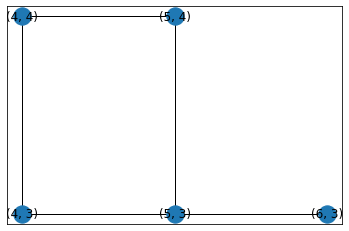Set up our combinations

We take the library of two-qubit circuits in circuit_library and mix-and-match to sampled in parallel.

We will pass combs_by_layer and circuit_library to the sampling function which will "zip" the circuits according to these combinations. The outer list corresponds to the four cirq.GridInteractionLayers (one of four for the degree-four GridQubit-implied graph). The inner combinations matrix is a (n_combinations, n_pairs) ndarray of integers which index into the circuit library.

combs_by_layer = rqcg.get_random_combinations_for_device(
n_library_circuits=len(circuit_library),
n_combinations=10,
device_graph=graph,
random_state=53,
)
combs_by_layer
[CircuitLibraryCombination(layer=cirq.experiments.GridInteractionLayer(col_offset=0, vertical=True, stagger=True), combinations=array([[ 5, 16],
[12,  9],
[ 5, 18],
[11,  3],
[ 6,  9],
[13,  3],
[11,  6],
[14, 12],
[16, 10],
[18, 15]]), pairs=[(cirq.GridQubit(4, 4), cirq.GridQubit(5, 4)), (cirq.GridQubit(5, 3), cirq.GridQubit(6, 3))]),
CircuitLibraryCombination(layer=cirq.experiments.GridInteractionLayer(col_offset=1, vertical=True, stagger=True), combinations=array([,
[ 3],
,
[ 0],
[ 5],
[ 5],
[ 0],
[ 7],
[ 9],
]), pairs=[(cirq.GridQubit(4, 3), cirq.GridQubit(5, 3))]),
CircuitLibraryCombination(layer=cirq.experiments.GridInteractionLayer(col_offset=1, vertical=False, stagger=True), combinations=array([,
[ 8],
[ 8],
,
[ 1],
,
,
[ 8],
,
]), pairs=[(cirq.GridQubit(4, 3), cirq.GridQubit(4, 4))]),
CircuitLibraryCombination(layer=cirq.experiments.GridInteractionLayer(col_offset=0, vertical=False, stagger=True), combinations=array([,
,
[ 2],
[ 6],
[ 1],
[ 9],
,
[ 1],
[ 9],
[ 6]]), pairs=[(cirq.GridQubit(5, 3), cirq.GridQubit(5, 4))])]

Visualize

Here, we draw the four layers' active pairs.

%matplotlib inline
from matplotlib import pyplot as plt

fig, axes = plt.subplots(2,2, figsize=(9,6))
for comb_layer, ax in zip(combs_by_layer, axes.reshape(-1)):
active_qubits = np.array(comb_layer.pairs).reshape(-1)
colors = ['red' if q in active_qubits else 'blue' for q in graph.nodes]
nx.draw_networkx(graph, pos=pos, node_color=colors, ax=ax)
nx.draw_networkx_edges(graph, pos=pos, edgelist=comb_layer.pairs, width=3, edge_color='red', ax=ax)

plt.tight_layout()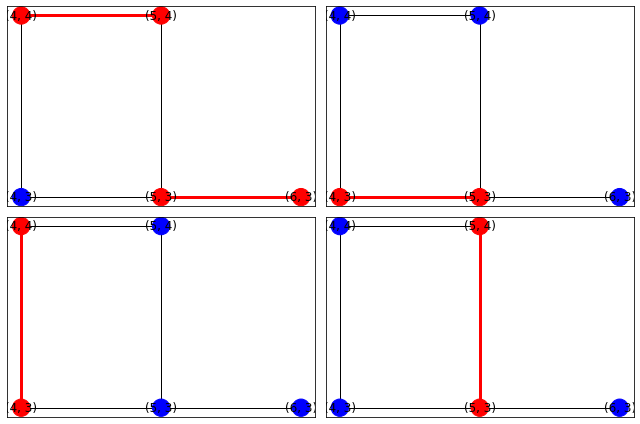Take Data

The following call will execute the zipped circuits and sample bitstrings.

from cirq.experiments.xeb_sampling import sample_2q_xeb_circuits
sampled_df = sample_2q_xeb_circuits(
sampler=sampler,
circuits=circuit_library,
cycle_depths=cycle_depths,
combinations_by_layer=combs_by_layer,
shuffle=np.random.RandomState(52),
repetitions=10_000,
)
sampled_df
100%|██████████| 207/207 [01:29<00:00,  2.32it/s]

Benchmark Fidelities

from cirq.experiments.xeb_fitting import benchmark_2q_xeb_fidelities
fids = benchmark_2q_xeb_fidelities(
sampled_df=sampled_df,
circuits=circuit_library,
cycle_depths=cycle_depths,
)
fids
from cirq.experiments.xeb_fitting import fit_exponential_decays, exponential_decay
fidelities = fit_exponential_decays(fids)
heatmap_data = {}

for (_, _, pair), fidelity in fidelities.layer_fid.items():
heatmap_data[pair] = 1.0 - fidelity

cirq.TwoQubitInteractionHeatmap(heatmap_data).plot();
(<AxesSubplot:xlabel='column', ylabel='row'>,
<matplotlib.collections.PolyCollection at 0x7f797c84a250>)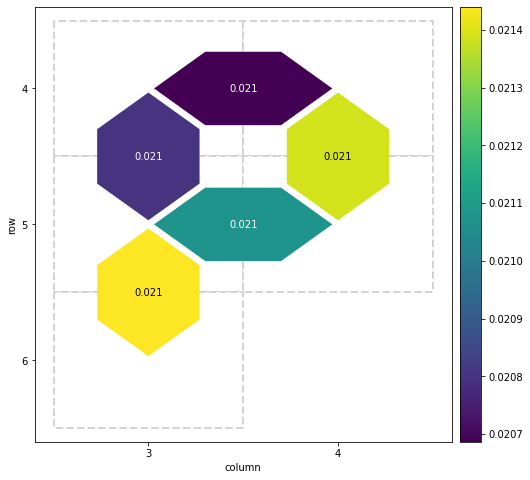for i, record in fidelities.iterrows():
plt.axhline(1, color='grey', ls='--')
plt.plot(record['cycle_depths'], record['fidelities'], 'o')
xx = np.linspace(0, np.max(record['cycle_depths']))
plt.plot(xx, exponential_decay(xx, a=record['a'], layer_fid=record['layer_fid']))
plt.show()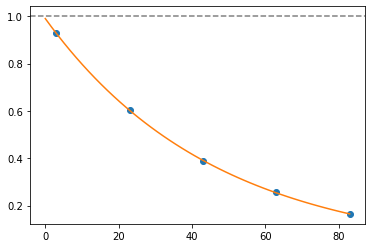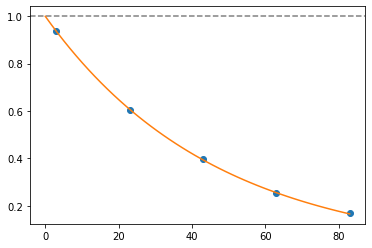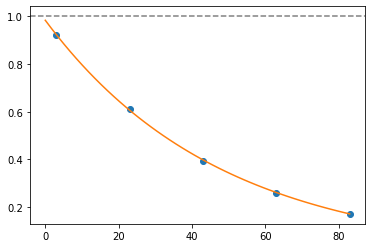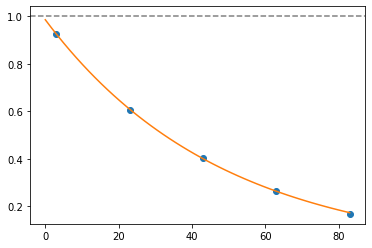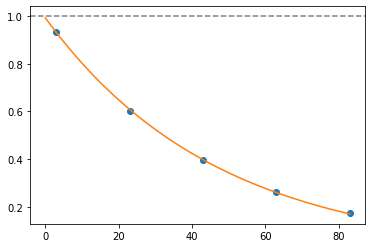import seaborn as sns

# Give each pair its own color
colors = sns.cubehelix_palette(n_colors=graph.number_of_edges())
colors = dict(zip(graph.edges, colors))

# Exponential reference
xx = np.linspace(0, fids['cycle_depth'].max())
plt.plot(xx, (1-5e-3)**(4*xx), label=r'Exponential Reference', color='black')

# Plot each pair
def _p(fids):
q0, q1 = fids.name
plt.plot(fids['cycle_depth'], fids['fidelity'],
'o-', label=f'{q0}-{q1}', color=colors[fids.name], alpha=0.5)
fids.groupby('pair').apply(_p)

plt.ylabel('Circuit fidelity')
plt.xlabel('Cycle Depth $d$')
plt.legend(loc='best')
plt.tight_layout()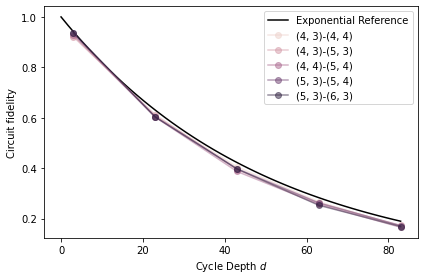Optimize PhasedFSimGate parameters

We know what circuits we requested, and in this simulated example, we know what coherent error has happened. But in a real experiment, there is likely unknown coherent error that you would like to characterize. Therefore, we make the five angles in PhasedFSimGate free parameters and use a classical optimizer to find which set of parameters best describes the data we collected from the noisy simulator (or device, if this was a real experiment).

import multiprocessing
pool = multiprocessing.get_context('spawn').Pool()
from cirq.experiments.xeb_fitting import (
parameterize_circuit,
characterize_phased_fsim_parameters_with_xeb_by_pair,
SqrtISwapXEBOptions,
)

# Set which angles we want to characterize (all)
options = SqrtISwapXEBOptions(
characterize_theta = True,
characterize_zeta = True,
characterize_chi = True,
characterize_gamma = True,
characterize_phi = True
)
# Parameterize the sqrt(iswap)s in our circuit library
pcircuits = [parameterize_circuit(circuit, options) for circuit in circuit_library]

# Run the characterization loop
characterization_result = characterize_phased_fsim_parameters_with_xeb_by_pair(
sampled_df,
pcircuits,
cycle_depths,
options,
pool=pool,
# ease tolerance so it converges faster:
fatol=1e-2,
xatol=1e-2
)
characterization_result.final_params
{(cirq.GridQubit(4, 3), cirq.GridQubit(5, 3)): {'theta': -0.7550388143989859,
'zeta': 0.03195237843993919,
'chi': -0.032390552955645455,
'gamma': 0.02487848987716369,
'phi': -0.05204064843025695},
(cirq.GridQubit(4, 3), cirq.GridQubit(4, 4)): {'theta': -0.8114255352566454,
'zeta': -0.07916161068034738,
'chi': -0.033322018406026034,
'gamma': 0.00731772516620779,
'phi': 0.04649794122241639},
(cirq.GridQubit(4, 4), cirq.GridQubit(5, 4)): {'theta': -0.7803560873231146,
'zeta': 0.03229968090224507,
'chi': -0.022495027919617255,
'gamma': 0.0028332950763749554,
'phi': 0.03710720169254639},
(cirq.GridQubit(5, 3), cirq.GridQubit(6, 3)): {'theta': -0.7584534353543917,
'zeta': 0.012197359358792513,
'chi': -0.05549817019216334,
'gamma': 0.0003480966933160427,
'phi': 0.026205488103712024},
(cirq.GridQubit(5, 3), cirq.GridQubit(5, 4)): {'theta': -0.8382301980930931,
'zeta': 0.10232624619886531,
'chi': -0.07597548049141974,
'gamma': -0.011239182878294323,
'phi': -0.004481745904481117} }
characterization_result.fidelities_df
from cirq.experiments.xeb_fitting import before_and_after_characterization
before_after_df = before_and_after_characterization(fids, characterization_result)
before_after_df
for i, row in before_after_df.iterrows():
plt.axhline(1, color='grey', ls='--')
plt.plot(row['cycle_depths_0'], row['fidelities_0'], '*', color='red')
plt.plot(row['cycle_depths_c'], row['fidelities_c'], 'o', color='blue')

xx = np.linspace(0, np.max(row['cycle_depths_0']))
plt.plot(xx, exponential_decay(xx, a=row['a_0'], layer_fid=row['layer_fid_0']),
color='red', label=f'f_0 = {row["layer_fid_0"]:.3f}')
plt.plot(xx, exponential_decay(xx, a=row['a_c'], layer_fid=row['layer_fid_c']),
color='blue', label=f'f_c = {row["layer_fid_c"]:.3f}')

plt.xlabel('Cycle Depth')
plt.ylabel('Fidelity')
plt.legend(loc='best')
plt.tight_layout()
plt.show()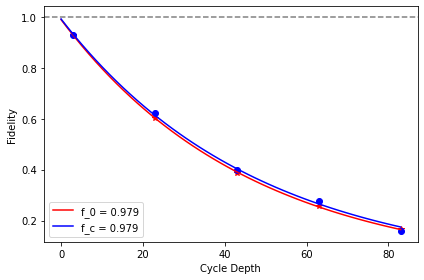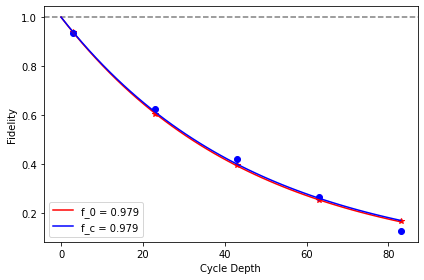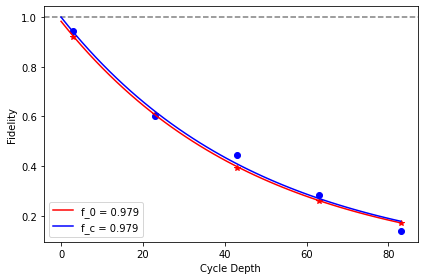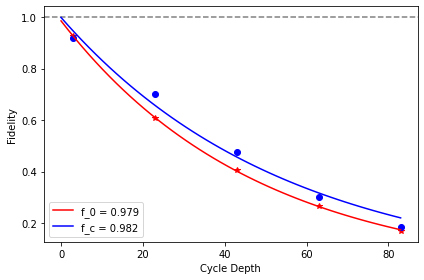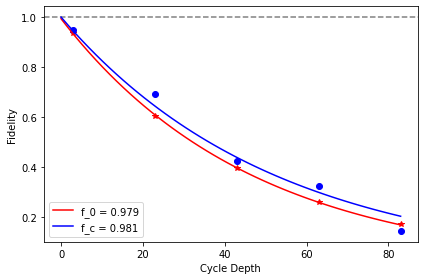[{ "type": "thumb-down", "id": "missingTheInformationINeed", "label":"Missing the information I need" },{ "type": "thumb-down", "id": "tooComplicatedTooManySteps", "label":"Too complicated / too many steps" },{ "type": "thumb-down", "id": "outOfDate", "label":"Out of date" },{ "type": "thumb-down", "id": "samplesCodeIssue", "label":"Samples / code issue" },{ "type": "thumb-down", "id": "otherDown", "label":"Other" }]
[{ "type": "thumb-up", "id": "easyToUnderstand", "label":"Easy to understand" },{ "type": "thumb-up", "id": "solvedMyProblem", "label":"Solved my problem" },{ "type": "thumb-up", "id": "otherUp", "label":"Other" }]